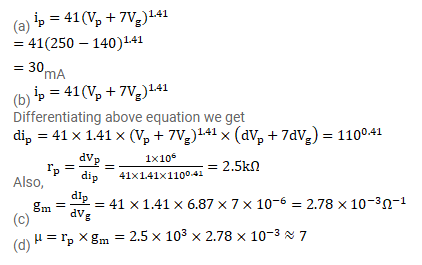# The plate current, plate voltage and grid voltage of

Question:

The plate current, plate voltage and grid voltage of a $6 \mathrm{~F} 6$ triode tube are related as $\mathrm{i}_{\mathrm{p}}=41\left(\mathrm{~V}_{\mathrm{p}}+7 \mathrm{~V}_{\mathrm{g}}\right)^{1.41}$

Where $v_{p}$ and $v_{g}$ are in volts and $i_{p}$ in microamperes. The tube is operated at $v_{p}=250 v_{1} v_{g}=-20 v$. Calculate

(a) the tube current,

(b) the plate resistance,

(c) the mutual conductance and

(d) the amplification factor.

Solution: